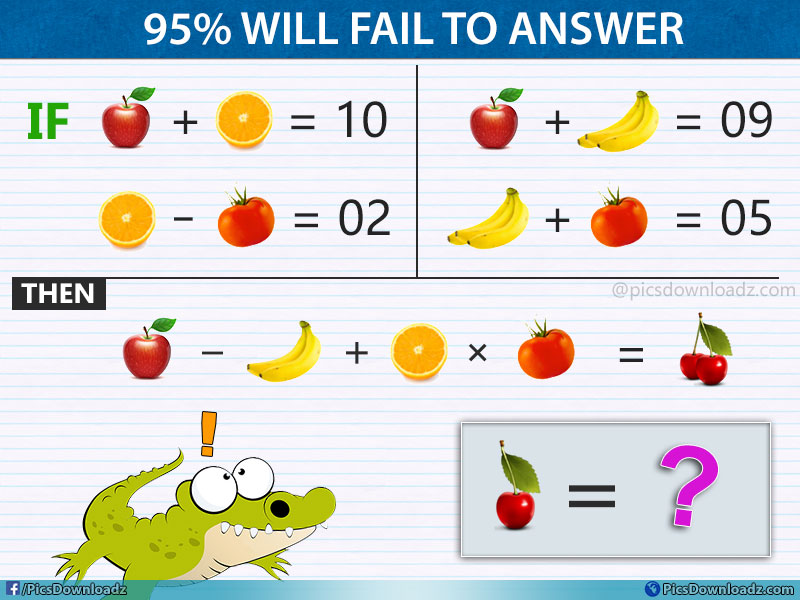This post may contain affiliate links. This means if you click on the link and purchase the item, We will receive an affiliate commission at no extra cost to you. See Our Affiliate Policy for more info.

The Apple, Banana, Orange and Tomato Puzzle – Viral Fruits Math Puzzle

0
3426

95% of people will fail to attempt this genius fruits puzzle. Solve this Only for Genius Puzzle Image. Fruits Math Puzzle with the correct answer!

The puzzle is divided into two parts. In the first part, there are the number of equations given in this puzzle, you have to find out the value of these fruits; Apple, Banana, Orange and Tomato and then solve the last equation.

Once you find the value of these fruits now put their value in the second part of the puzzle and find the correct value of Cherry. Find out which number will replace the question mark?

Not as hard as you are thinking, just twist little bit and you will able to solve this puzzle easily. This puzzle will test your basic math skill and IQ level. Take your time, the comment section is all yours. Let see how many of you can crack this genius fruits puzzle. Good luck!

Question:

IF:

1. Apple + Orange = 10
2. Orange – Tomato = 02
3. Apple + Banana = 09
4. Banana + Tomato = 05

Then:

1. Apple – Banana + Orange × Tomato = Cherry

Find the value of “Cherry”

Fruits Math Puzzle Image:[Embed Code for this Image given below]

Share you answer below or keep scrolling for the correct answer and solution.

.

A

N

S

W

E

R

.

Solution:

#1st Part of the puzzle:

Given Equations are:

1. Apple + Orange = 10
2. Orange – Tomato = 02
3. Apple + Banana = 09
4. Banana + Tomato = 05

Compare equation 2 and 4, we will get

⇒ Orange – Tomato + Banana + Tomato = 2 + 5

⇒ Orange + Banana = 7   (called it eq. 6)

Now from equation 1

⇒ Apple + Orange = 10

We can write this equation as follow: Apple = 10 – Orange   (Put Apple value in equation 3)

Equation 3:

⇒ Apple + Banana = 09

⇒ 10 – Orange + Banana = 09

⇒ – Orange + Banana = 9 – 10

⇒ – Orange + Banana = –1    (called it eq. 7)

Compare Eq. 6 & 7; we will get

⇒ Orange + Banana – Orange + Banana = 7 – 1

⇒ 2(Banana) = 6

⇒ Banana = 6/2 = 3

⇒ Banana = 3

Now in Eq. 3:

⇒ Apple + Banana = 9

⇒ Apple + 3 = 9

⇒ Apple = 9 – 3

⇒ Apple = 6

In Eq. 4:

⇒ Banana + Tomato = 5

⇒ 3 + Tomato = 5

⇒ Tomato = 5 – 3

⇒ Tomato = 2

In Eq. 1:

⇒ Apple + Orange = 10

⇒ 6 + Orange = 10

⇒ Orange = 10 – 6

⇒ Orange = 4

#2nd Part of the puzzle:

⇒ Apple – Banana + Orange × Tomato = Cherry

We have all fruits (& vegetable) value as follow:

Apple = 6, Orange = 4, Banana = 3 and Tomato = 2

Now if you noticed, in the first part of the puzzle there are three banana in the equation which equals to 3, which means each banana has one value

i.e Banana (Three) = 3  and Banana (Two) = 2

Our Last equation i.e. 5th is:

⇒ Apple – Banana (Two) + Orange × Tomato = Cherry

Now, put their values in the equation

⇒ 6 – 2 + 4 * 2 = ? (Acc. To Order of operation, multiplication first then add and sub.)

⇒ 6 – 2 + 8 = ?

⇒ 4 + 8 = ?

⇒ 4 + 8 = 12

⇒ Cherries = 12

There are Two Cherries in the second part of the puzzle but we will have to find out the value of only one cherry (as you can see in the puzzle image)

Therefore 2 Cherries = 12

So, One Cherry = 12/2 = 6 Correct Answer

Hope, you found this puzzle interesting. 😉

Like our Page: